# Types of Comparator

Comparator is a part of static relay, which receives two or more inputs to be compared and gives output based on the comparison.

# Types of Comparator

The various types of comparators are;
1. Amplitude Comparator
2. Phase Comparator
3. Hybrid Comparator

### Amplitude Comparator

An amplitude comparator compares the magnitude of two input quantities irrespective of the angle between them. One of the inputs is the operating quantity and the other a restraining quantity.

When the amplitude of the operating quantity exceeds that of restraining quantity, the relay sends a tripping signal to the circuit breaker.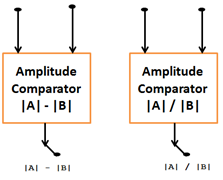- The Amplitude comparator compares two vector, |A| and |B|
- Gives an output: the algebraic difference between the magnitudes |A| and |B|
- Output is +ve, if |A| > |B|
- Output is –ve, if |A| < |B|
- Output is zero, if |A| = |B|

Comparison by ratio:
- Output is >1, if |A| > |B|
- Output <1, if |A| < |B|
- Output is Zero, if |A| is zero.

## Amplitude types of comparator.

1. Integrating comparator
2. Instantaneous comparator
3. Sampling Comparator

### Integrating Comparator

- Circulating Current Type
- Opposed Voltage Type

#### Circulating Current Type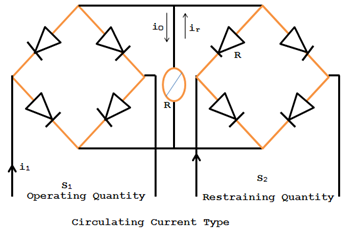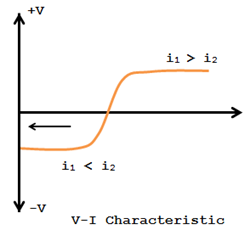It can also be used as impedance relay. Two rectifier  bridges can be arranged in such a manner as shown in the figure below, to function as amplitude comparator circulating type.

The polarized relay operates when S1>S2 where S1=K1i1 and S2 = K2i2. This arrangement gives a sensitive relay whose voltage may be represented in the VI characteristic of the figure.

#### Opposed Voltage typeThis type works with voltage input signals derived from PTs. The operation depends on the difference of the average rectified voltage (V1-V2).Here the rectifiers are not protected against higher currents. The relay operates when V1 >V2.

#### Instantaneous Comparator (Directing Amplitude Comparator) – Averaging Type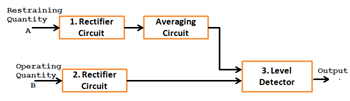Here the restraining signal is rectified and smoothed completely in order to provide a level restraint.

This is then compared with the peak value of operating signal, which may or may not be rectified but is smoothened. The tripping signal is provided if the operating signal exceeds the level of the restraint. The block diagram is shown in the fig above. Since this method involves smoothening, the operation is slow. A faster method is phase splitting the wave shapes of instantaneous amplitude comparator are shown in fig below before rectification and the averaging circuit can be eliminated.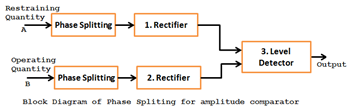#### Phase Comparator

Phase comparison technique is the most widely used one for all practical directional, distance, differential and carrier relays.

If the two input signals are S1 and S2 the output occurs when the inputs have phase relationship lying within the specified limits.

Both the input must exist for an output to occur. The operation is independent of their magnitudes and is dependent only on their phase relationship. The figures below show that the phase comparator is simple form. The function is defined by the boundary of marginal operation and represented by the straight lines from the origin of the S-plane.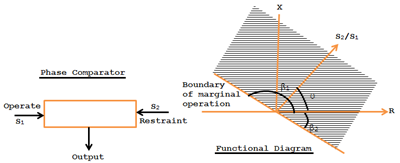The condition of operation is β1 < θ < β2.

θ is the angle by which S2 lands S1. If β1 = β2 =90o, the comparator is called cosine comparator and if β1=0 and β2=180o, it is a sine comparator.

In short, a phase comparator compares two input quantities in phase angle (vertically) irrespective of the magnitude and operates if the phase angle between them is < 90o.

There are two types of phase comparators:
1. Vector product comparator
2. Coincidence type phase comparator.

#### Vector Product comparator

This comparator recognizes the vector product or division between the two or more quantities. Thus, the output is A, B or A/B

#### Coincidence Comparator

Consider two signals S1 and S2. The period of Coincidence of S1 and S2 will depend on the phase difference between S1 and S2. The fig below shows the coincidence of S1 and S2 when S2 lags S1 by less than π/2 ie., θ.

The period of coincidence of S1 and S2 with a phase difference of θ is Ψ = 180o – θ. Different techniques are used to measure the period of coincidence. Two of the important types are

1. Bloke Spike Method (Direct Phase Comparison) and
2. Coincidence type – Integrating phase comparator.

### Hybrid Comparator

This kind of comparator compares both magnitude and phase of the input quantities. Hence this type is of mixed version.

In the hybrid comparator, both amplitude and phase comparators are used. Inputs are given to a phase comparator. The output of the phase comparator is given to amplitude comparator.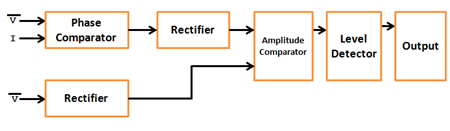Static impedance relays comparing V and I are generally of Hybrid Comparator.

### Level Detector

Level detector determines the level of its input with reference to a predetermined setting.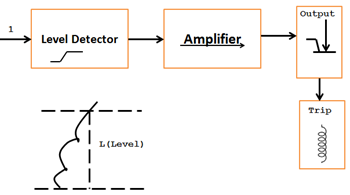When input I exceed the level (L) and the output (O) of the level detectors exceeds and the output stage of the relays gets a triggering signal via an amplifier.0 CommentsComments